Author Message
LiquidCode
Moderator Team

Joined: 05 Dec 2000
Posts: 1736
Location: Space and TimePosted: Fri Oct 21, 2011 9:50 pm    Post subject: FindInINI function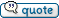This is a function that I created for a program and I thought I would share. It locates items in an ini file when not all of the info is known.

Take the following for example:

[user_000]
name=Mr. Foo
email=MF1245@me.com

[user_001]
Name=John Pocket
email=Mr.JP@me.com

This file was generated by an online company. One section per user with info. I know the user's email address but not the name and the section (user number).

I would use my function to find the section the email address is in then I can find the user's name.

%t = @FindInINI(@path(%0)MyIni.ini,,,MF1245@me.com)

This would return User_000|email.... <Section>|<Key>
I can parse it to get the section and then use @iniread(User_000,name) to get the user's name.

Anyway, here is the code.

 Code: :FindInINI   #%a = @FindInINI(,
,,[,)   #this will close any previously opened ini file and open the file provided.   #this is used to find a Key or Value in an ini file.   #if a Section and key are used. The value is returned just like @iniread(section,key)   #if only a Section and value is used, that whole section is searched and   #returns the KEY name it was found under.   #if no Section supplied then the whole file is searched.   #if a key name is supplied, the file is searched for the first matching   #key and returns Section|value   #if only the value is supplied then the whole file is searched and returns Section|key   if @not(%1)     error 2   end   if @not(%3)     if @not(%4)       error 2     end   end   inifile open,%1   %%find_in = @new(list)   if %2     list assign,%%Find_in,@iniread(%2)     if @not(@text(%%Find_in))       %%find_exit =     end     if %3       %%find_exit = @iniread(%2,%3)     elsif %4       %x = 0       repeat         %i = @item(%%Find_In,%x)         if @greater(@pos("=",%i),0)           %%find_key = @trim(@substr(%i,1,@pred(@pos("=",%i))))           %%find_value = @trim(@substr(%i,@succ(@pos("=",%i)),@len(%i)))           if @equal(%4,%%Find_Key)             %%find_exit = %%Find_Key             %x = @pred(@count(%%Find_In))           end         end         %x = @succ(%x)       until @equal(%x,@count(%%Find_In))     else       %%find_exit =     end   else     list assign,%%Find_in,@iniread()     %x = 0     repeat       %i = @item(%%Find_In,%x)       if %3         %t = @iniread(%i,%3)         if %t           if @equal(%5,s)             %%find_exit = %i           elsif @equal(%5,k)             %%find_exit = %t           else             %%find_exit = %i|%t           end           %x = @pred(@count(%%Find_In))         end       elsif %4         %%find_in2 = @new(list)         list assign,%%Find_In2,@iniread(%i)         %y = 0         repeat           %j = @item(%%Find_in2,%y)           if @greater(@pos("=",%j),0)             %%find_key = @trim(@substr(%j,1,@pred(@pos("=",%j))))             %%find_value = @trim(@substr(%j,@succ(@pos("=",%j)),@len(%j)))             if @equal(%4,%%Find_Value)               if @equal(%5,s)                 %%find_exit = %i               elsif @equal(%5,k)                 %%find_exit = %%Find_Key               else                 %%find_exit = %i|%%Find_Key               end               %y = @pred(@count(%%Find_In2))             end           end           %y = @succ(%y)         until @equal(%y,@count(%%Find_in2))         if %%Find_Exit           %x = @pred(@count(%%Find_In))         end         list close,%%find_in2       end       %x = @succ(%x)     until @equal(%x,@count(%%Find_in))   end   inifile close   list close,%%Find_in   exit %%Find_exit

_________________
Chris
Http://vdsfreak.com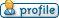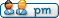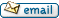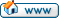Display posts from previous: All Posts1 Day7 Days2 Weeks1 Month3 Months6 Months1 Year Oldest FirstNewest First
 All times are GMT Page 1 of 1